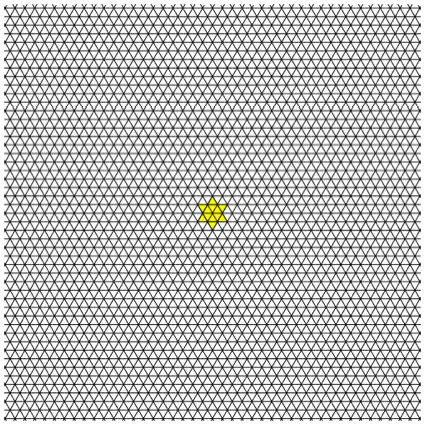Hans Walser, [20090701a]

The Fibonacci Star

# 1        The StarThe Fibonacci Star

# 2        How to get it

We work in the regular triangular lattice, using so called Fibonacci trapezoids.Fibonacci Trapezoids

These are isosceles trapezoids with top length, sides of length, and a base length.Fibonacci Recursion

# 3        Sequence of FiguresInitial hexagonHexagonal star in the centreThe first trapezoidsNext stepNext ring of trapezoidsA further ringHow does it go on?